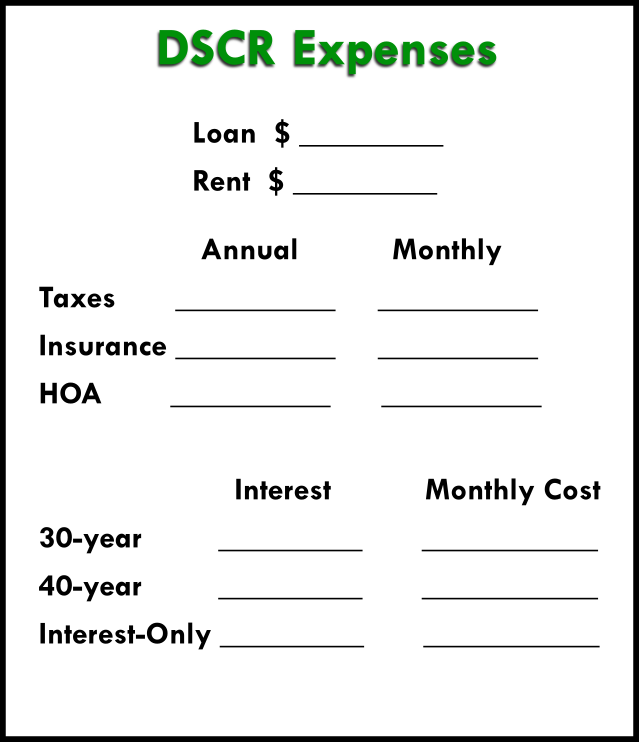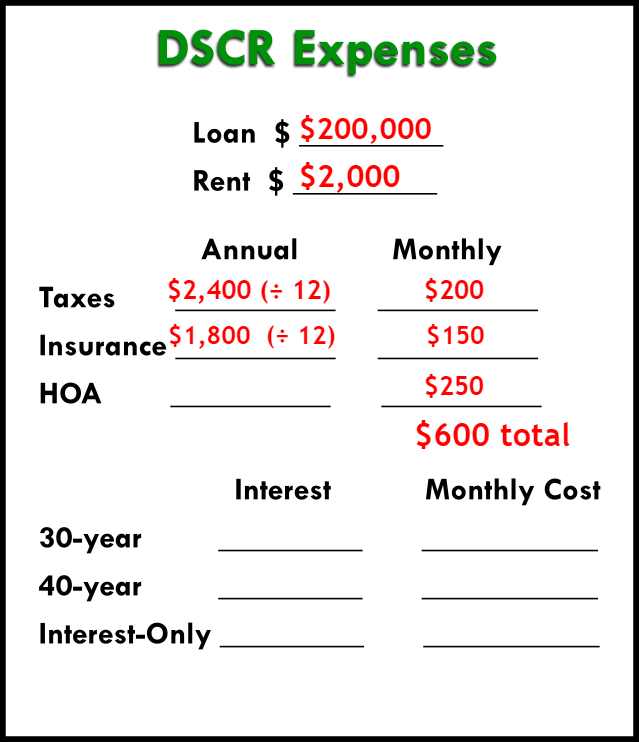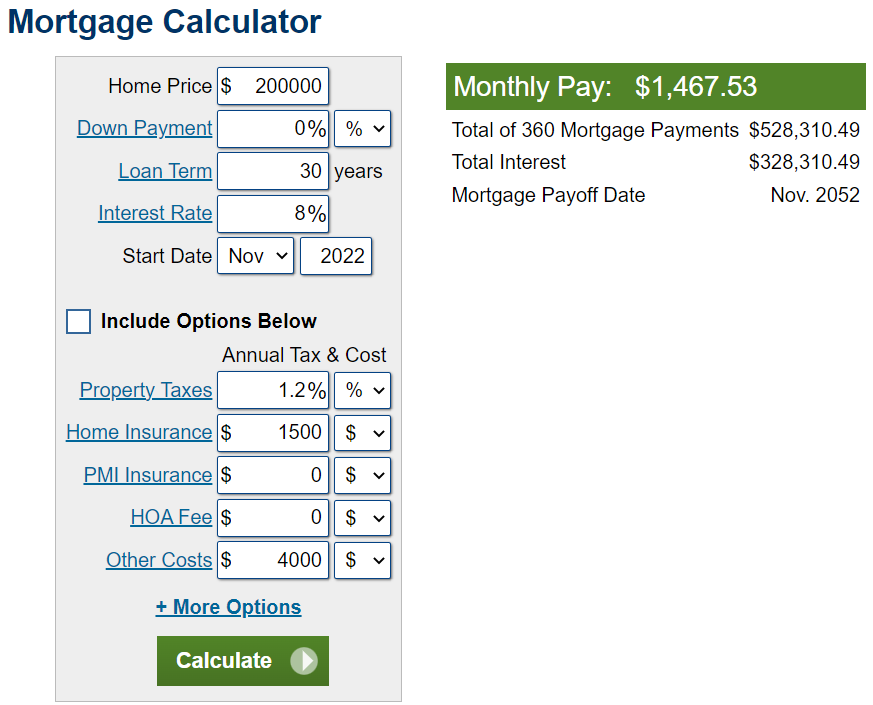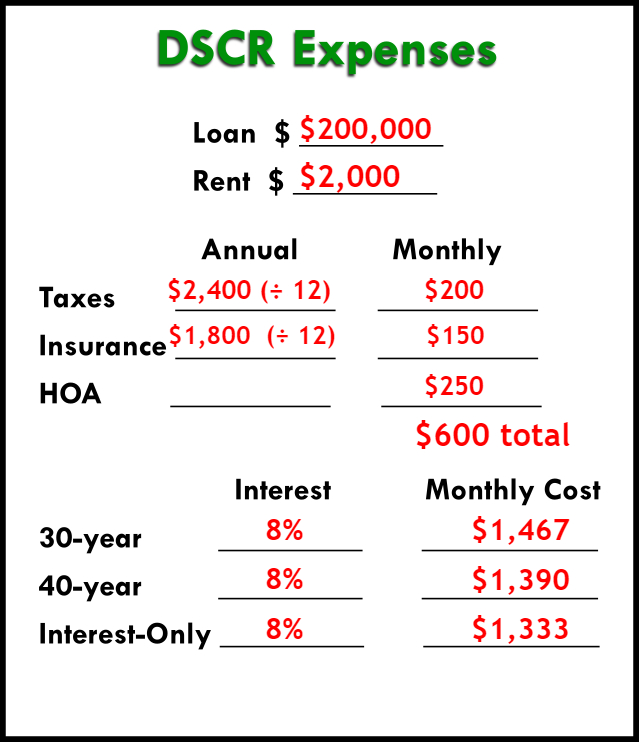# Sample of a DSCR Loan Calculation: The Costs

Categories:

An important part of a DSCR loan is knowing your costs. Here’s an example DSCR loan calculation to help you out.

A DSCR loan will be a useful product in your real estate investing career. It requires no income verification and no work or investment history. These loans only require that the property’s income is the same (or higher than) the expenses.

Cash flow really is kind for DSCR loans. Here’s a breakdown of how to calculate the expenses to see if you qualify.

## Calculate a DSCR Loan Expenses

You can follow along with your DSCR loan calculator (free download here). We’ll fill out this form to show each step of a DSCR loan calculation.### Rent Income & Loan Amount

Firstly, you need to estimate your loan amount and your rent income. If you have a deal in front of you, you probably have a good idea of the loan amount you’ll need to be able to afford the property.

As for rent, you can get realistic amounts from online sources. Look at Zillow or Rent.com to find the market rate for rent in the property’s neighborhood.

Let’s keep it simple for our example and say our loan is \$200,000, and our rent income is \$2,000.

### What Expenses Count in a DSCR Loan?

We know our income (rent), but now we need to figure out our costs.

The expenses considered in a DSCR loan DO include:

Taxes

Insurance

HOA fees

Expenses NOT considered in a DSCR loan are things like:

Property management fees

Utilities

Maintenance

To estimate the taxes on the property, you could use a property tax calculator like this one. If you need an estimate on insurance, you can try this home insurance calculator. You can figure out HOA fees by contacting the HOA, if that applies to your property.

If any of these costs are charged annually, then you’ll need to divide by 12 to break it down into a monthly cost.

Let’s take a look at what information we have now for our example DSCR loan:### Calculating Loan Cost

Secondly, DSCR lenders will offer many types of the loans – fixed-rate mortgages, interest-only, ARMs, etc. You need to find what best fits you, and to do that, you’ll have to run all the numbers.

To calculate each of the amortized loans, you can use an amortization calculator like this one. Add in your information – loan amount, interest rate, and loan length.We’re going to use an 8% interest rate for our example, since that’s the anticipated average for next year.

In reality, each loan and lender will have a different interest rate. Additionally, the interest rate may fluctuate depending on your qualifications and DSCR. You can get this information from your lenders to plug into your calculator.

We’ll use three common loans for this example: a 30-year fixed, 40-year fixed, and interest only loan.

If we had a 30-year mortgage for \$200,000 at 8%, our monthly payment would be \$1,467.

For a 40-year fixed with the same info, payments would be \$1,390.

For interest-only, you can calculate the loan fairly simply yourself. Multiply the loan amount by the interest rate (e.g., 200,000 × .08 = 16,000). That gives you the yearly interest, then you divide it by 12 to get the monthly payment. For our example, that’s \$1,333.So what do you do with these numbers? How do you know which loan is best?

It depends on your priorities. To have the most cash flow, the lowest number is best (in this case, interest-only). If you need something that amortizes, a 30-year would probably be best.

But you don’t really know which loan will be best for you until you calculate the DSCR.

Watch the video here.

by